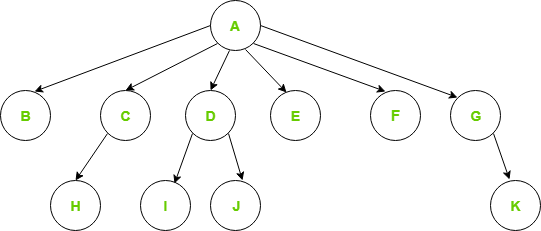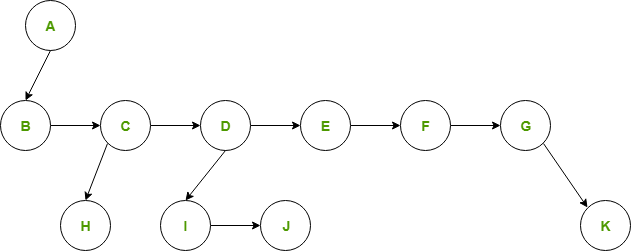# Introduction to Generic Trees (N-ary Trees)

Generic trees are a collection of nodes where each node is a data structure that consists of records and a list of references to its children(duplicate references are not allowed). Unlike the linked list, each node stores the address of multiple nodes. Every node stores address of its children and the very first node’s address will be stored in a separate pointer called root.

The Generic trees are the N-ary trees which have the following properties:

1. Many children at every node.

2. The number of nodes for each node is not known in advance.

Example:Generic Tree

To represent the above tree, we have to consider the worst case, that is the node with maximum children (in above example, 6 children) and allocate that many pointers for each node.
The node representation based on this method can be written as:

## C++

 `// Node declaration ` `struct` `Node{ ` `   ``int` `data; ` `   ``Node *firstchild; ` `   ``Node *secondchild; ` `   ``Node *thirdchild; ` `   ``Node *fourthchild; ` `   ``Node *fifthchild; ` `   ``Node *sixthchild; ` `};`

## C

 `//Node declaration ` `struct` `Node{ ` `   ``int` `data; ` `   ``struct` `Node *firstchild; ` `   ``struct` `Node *secondchild; ` `   ``struct` `Node *thirdchild; ` `   ``struct` `Node *fourthchild; ` `   ``struct` `Node *fifthchild; ` `   ``struct` `Node *sixthchild; ` `} `

## Java

 `// Java code for above approach ` `public` `class` `Node { ` `    ``int` `data; ` `    ``Node firstchild; ` `    ``Node secondchild; ` `    ``Node thirdchild; ` `    ``Node fourthchild; ` `    ``Node fifthchild; ` `    ``Node sixthchild; ` `}`

## Python

 `class` `Node: ` `    ``def` `__init__(``self``, data): ` `        ``self``.data ``=` `data ` `        ``self``.firstchild ``=` `None` `        ``self``.secondchild ``=` `None` `        ``self``.thirdchild ``=` `None` `        ``self``.fourthchild ``=` `None` `        ``self``.fifthchild ``=` `None` `        ``self``.sixthchild ``=` `None`

## C#

 `public` `class` `Node ` `{ ` `    ``public` `int` `Data { ``get``; ``set``; } ` `    ``public` `Node Firstchild { ``get``; ``set``; } ` `    ``public` `Node Secondchild { ``get``; ``set``; } ` `    ``public` `Node Thirdchild { ``get``; ``set``; } ` `    ``public` `Node Fourthchild { ``get``; ``set``; } ` `    ``public` `Node Fifthchild { ``get``; ``set``; } ` `    ``public` `Node Sixthchild { ``get``; ``set``; } ` `}`

## Javascript

 `// Javascript code for above approach ` `class Node { ` `    ``constructor(data) { ` `        ``this``.data = data; ` `        ``this``.firstchild = ``null``; ` `        ``this``.secondchild = ``null``; ` `        ``this``.thirdchild = ``null``; ` `        ``this``.fourthchild = ``null``; ` `        ``this``.fifthchild = ``null``; ` `        ``this``.sixthchild = ``null``; ` `    ``} ` `}`

Disadvantages of the above representation are:

1. Memory Wastage – All the pointers are not required in all the cases. Hence, there is lot of memory wastage.
2. Unknown number of children – The number of children for each node is not known in advance.

Simple Approach:

For storing the address of children in a node we can use an array or linked list. But we will face some issues with both of them.

1. In Linked list, we can not randomly access any child’s address. So it will be expensive.
2. In array, we can randomly access the address of any child, but we can store only fixed number of children’s addresses in it.

Better Approach:

We can use Dynamic Arrays for storing the address of children. We can randomly access any child’s address and the size of the vector is also not fixed.

## C++

 `#include ` ` `  `class` `Node { ` `public``: ` `    ``int` `data; ` `    ``std::vector children; ` ` `  `    ``Node(``int` `data) ` `    ``{ ` `        ``this``->data = data; ` `    ``} ` `}; `

## C

 `//Node declaration ` `struct` `Node{ ` `    ``int` `data; ` `    ``vector children; ` `} `

## Java

 `import` `java.util.ArrayList; ` ` `  `class` `Node { ` `    ``int` `data; ` `    ``ArrayList children; ` ` `  `    ``Node(``int` `data) ` `    ``{ ` `        ``this``.data = data; ` `        ``this``.children = ``new` `ArrayList(); ` `    ``} ` `}`

## Python

 `class` `Node: ` `     `  `    ``def` `__init__(``self``,data): ` `        ``self``.data``=``data ` `        ``self``.children``=``[]`

## C#

 `using` `System.Collections.Generic; ` ` `  `class` `Node { ` `    ``public` `int` `data; ` `    ``public` `List children; ` ` `  `    ``public` `Node(``int` `data) ` `    ``{ ` `        ``this``.data = data; ` `        ``this``.children = ``new` `List(); ` `    ``} ` `} ` ` `  `// This code is contributed by adityamaharshi21.`

## Javascript

 `class Node { ` `  ``constructor(data) { ` `    ``this``.data = data; ` `    ``this``.children = []; ` `  ``} ` `} `

#### Efficient Approach:

First child / Next sibling representation

In the first child/next sibling representation, the steps taken are:

At each node-link the children of the same parent(siblings) from left to right.

• Remove the links from parent to all children except the first child.

Since we have a link between children, we do not need extra links from parents to all the children. This representation allows us to traverse all the elements by starting at the first child of the parent.FIRST CHILD/NEXT SIBLING REPRESENTATION

The node declaration for first child / next sibling representation can be written as:

## C++

 `struct` `Node { ` `    ``int` `data; ` `    ``Node *firstChild; ` `    ``Node *nextSibling; ` `}; `

## C

 `//Node declaration ` `struct` `Node{ ` `    ``int` `data; ` `    ``struct` `Node *firstChild; ` `    ``struct` `Node *nextSibling; ` `} `

## Java

 `class` `Node { ` `    ``int` `data; ` `    ``Node firstChild; ` `    ``Node nextSibling; ` `} `

## Python

 `class` `Node: ` `    ``def` `__init__(``self``, data): ` `        ``self``.data ``=` `data ` `        ``self``.firstChild ``=` `None` `        ``self``.nextSibling ``=` `None` ` `  `        ``# This code is contributed by aadityamaharshi`

## Javascript

 `class Node { ` `    ``constructor(data) { ` `        ``this``.data = data; ` `        ``this``.firstChild = ``null``; ` `        ``this``.nextSibling = ``null``; ` `    ``} ` `} `

## C#

 `public` `class` `Node { ` `    ``public` `int` `Data ` `    ``{ ` `        ``get``; ` `        ``set``; ` `    ``} ` `    ``public` `Node FirstChild ` `    ``{ ` `        ``get``; ` `        ``set``; ` `    ``} ` `    ``public` `Node NextSibling ` `    ``{ ` `        ``get``; ` `        ``set``; ` `    ``} ` `}`